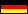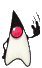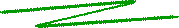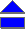The order ordn(m) of an integer m modulo nIf you exponentiate an integer number modulo another one, you may observe curious effects. For instance, 33 = 27 = 12 mod 15, whereas 34 = 81 = 6 mod 15. That is, multiplying 12 by 3 modulo 15 yields a number smaller than 12!? Or, if you calculate modulo 24 (the number of hours of a day), you obtain 33 = 27 = 3 mod 24. Funny, uh?

For some numbers m > 1 you even can find a power r such that mr = 1 mod n. For example we have 42 = 16 = 1 mod 15.

The order of an integer m modulo a (natural) number n is defined to be the smallest positive integer power r such that

mr = 1 mod n.

The order r of m modulo n is shortly denoted by ordn(m). For some constellations, however, there does not exists any positive power. Above we saw, e.g., that 33 = 3 mod 24, i.e., 33 = 31 mod 24, and moreover we directly compute 32 = 34 = 9 mod 24. Hence, any even power of 3 yields 9 modulo 24, and any odd power of 3 is 3 modulo 24.

Even worse, sometimes there are numbers with a positive power that vanishes. Look for example at 122 = 144 = 0 mod 24. For such numbers there does not exist a positive finite power to yield its order, and the order is then defined as infinite.

It is an important group theoretic result that if n is prime, then any integer relatively prime to n has a finite order. For instance if n = 7, the order of any number m can be read from the following table.

 m ord7(m) 1 2 3 4 5 6 8 ... 1 3 6 3 6 2 1 ...

The dots (...) represent the fact that anything repeats cyclically. Check it out! More generally, any number m being prime relatively to n has a finite order with respect to n, e.g., for n=15,

 m ord15(m) 1 2 4 7 8 11 13 14 1 4 2 4 4 2 4 2

In particular, the possible finite orders modulo n are divisors of the Carmichael function value, and vice versa any divisor of it is the order of at least one integer. For 15, the Carmichael function yields 4. In fact, all divisors of 4 (namely 1, 2, and 4) can be found as order values in the table above! You may call an appletwhich computes the order.

 © de Vries 2002 – 2012